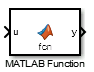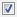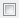# MATLAB Function

Include MATLAB code in models that generate embeddable C code

• Library:

HDL Coder / User-Defined Functions

•## Description

With a MATLAB Function block, you can write a MATLAB® function for use in a Simulink® model. The MATLAB function executes for simulation and generates code for a Simulink Coder™ target. If you are new to Simulink and MATLAB products, see Implementing MATLAB Functions Using Blocks for an overview.

Double-clicking the MATLAB Function block opens the MATLAB Function Block Editor, where you write the MATLAB function. The example model `call_stats_block2` discussed in Create Custom Functionality Using MATLAB Function Blocks uses the following function in the MATLAB Function Block Editor:

```function [mean,stdev] = stats(vals) % Calculates a statistical mean and a standard % deviation for the values in vals. len = length(vals); mean = avg(vals,len); stdev = sqrt(sum(((vals-avg(vals,len)).^2))/len); plot(vals,'-+'); function mean = avg(array,size) mean = sum(array)/size;```

The function specifies the input and output data in the function declaration statement as arguments and return values. The argument and return values of the preceding example function correspond to the inputs and outputs of the block in the `call_stats_block2` model.You can also define data, add an input trigger, and create function call outputs using the Model Explorer or the Ports and Data Manager. You can access the Ports and Data Manager in the MATLAB Function Block Editor by clicking Edit Data. For more information, see Define MATLAB Function Block Data, Manage the Input Trigger of a MATLAB Function Block, and Manage Function Call Outputs of a MATLAB Function Block.

The MATLAB Function block generates efficient embeddable code based on an analysis that determines the size, class, and complexity of each variable. This analysis imposes the following restrictions:

• The first assignment to a variable defines its, size, class, and complexity.

• You cannot reassign variable properties after the initial assignment except when using variable-size data or reusing variables in the code for different purposes.

In addition to language restrictions, the MATLAB Function block supports a subset of the functions available in MATLAB. These functions include functions in common categories, such as:

For more information, see Functions and Objects Supported for C/C++ Code Generation.

Note

Although the code for this block attempts to produce exactly the same results as MATLAB, differences might occur due to rounding errors. These numerical differences, which might be a few `eps` initially, can magnify after repeated operations. Reliance on the behavior of `nan` is not recommended. Different C compilers can yield different results for the same computation.

Note

New MATLAB Function blocks do not include the `%#codegen` directive, but check for errors as if it is included. Adding the `%#codegen` directive to a MATLAB Function block does not affect error checking. For more information see Compilation Directive %#codegen.

To support visualization of data, the MATLAB Function block supports calls to MATLAB functions for simulation only. See Use MATLAB Engine to Execute a Function Call in Generated Code to understand some of the limitations of this capability, and how it integrates with code analysis for this block. If these function calls do not directly affect any of the Simulink inputs or outputs, the calls do not appear in Simulink Coder generated code.

From MATLAB Function blocks, you can also call functions defined in a Simulink Function block. You can call Stateflow® functions with Export Chart Level Functions (Make Global) and Allow exported functions to be called by Simulink checked in the chart Properties dialog box.

In the Ports and Data Manager, you can declare a block input to be a Simulink parameter instead of a port. The MATLAB Function block also supports inheritance of types and size for inputs, outputs, and parameters. You can also specify these properties explicitly. See Define and Modify Function Argument Types, Specify Size of MATLAB Function Block Data, and Configure MATLAB Function Block Parameter Data Arguments for descriptions of variables that you use in MATLAB Function blocks.

Recursive calls are not allowed in MATLAB Function blocks.

By default, MATLAB Function blocks have direct feedthrough enabled. To disable it, clear the Allow direct feedthrough property. Nondirect feedthrough semantics ensure that outputs rely only on the current state. Using nondirect feedthrough enables you to use MATLAB Function blocks in a feedback loop and prevent algebraic loops. For more information, see Use Nondirect Feedthrough in a MATLAB Function Block.

## Ports

### Input

expand all

Input corresponding to the first input argument of the function inside the MATLAB Function block. If you rename the function argument in the editor, the block renames the port correspondingly.

Data types supported by MATLAB but not supported by Simulink may not be passed between the Simulink model and the function within the MATLAB Function block. These types may be used within the MATLAB Function block.

For more information on fixed-point support for this block, refer to Fixed-Point Data Types with MATLAB Function Block (Fixed-Point Designer) and MATLAB Function Block with Data Type Override (Fixed-Point Designer).

Data Types: `single` | `double` | `int8` | `int16` | `int32` | `int64` | `uint8` | `uint16` | `uint32` | `uint64` | `Boolean` | `string` | `fixed point` | `enumerated` | `bus`

`n`th input argument to the function in the MATLAB Function block. When you add the argument in the editor, the block adds the port correspondingly.

Data types supported by MATLAB but not supported by Simulink may not be passed between the Simulink model and the function within the MATLAB Function block. These types may be used within the MATLAB Function block.

For more information on fixed-point support for this block, refer to Fixed-Point Data Types with MATLAB Function Block (Fixed-Point Designer) and MATLAB Function Block with Data Type Override (Fixed-Point Designer).

Data Types: `single` | `double` | `int8` | `int16` | `int32` | `int64` | `uint8` | `uint16` | `uint32` | `uint64` | `Boolean` | `string` | `fixed point` | `enumerated` | `bus`

### Output

expand all

First output argument of the function inside the MATLAB Function block. If you rename the function argument in the editor, the block renames the port correspondingly.

Data Types: `single` | `double` | `int8` | `int16` | `int32` | `int64` | `uint8` | `uint16` | `uint32` | `uint64` | `Boolean` | `string` | `fixed point` | `enumerated` | `bus`

Nth output argument from the function inside the MATLAB Function block. When you add the argument in the editor, the block adds the port correspondingly.

Data Types: `single` | `double` | `int8` | `int16` | `int32` | `int64` | `uint8` | `uint16` | `uint32` | `uint64` | `Boolean` | `string` | `fixed point` | `enumerated` | `bus`

## Parameters

expand all

Parameters in the Code Generation tab require Simulink Coder or Embedded Coder®.

### Main

Select how to display port labels on the MATLAB Function block icon.

`none`

Do not display port labels.

`FromPortIcon`

Display the name of the function input and output arguments.

`FromPortBlockName`

Display the name of the function input and output arguments.

`SignalName`

If the signal connected to the port is named, display the signal name. Otherwise, display the name of the function argument.

#### Programmatic Use

 Parameter: `ShowPortLabels` Type: character vector Value: `'FromPortIcon'` | `'FromPortBlockName'` | `'SignalName'` Default: `'FromPortIcon'`

`ReadWrite`

Enable opening and modification of MATLAB Function block contents.

`ReadOnly`

Enable opening but not modification of the MATLAB Function block.

`NoReadOrWrite`

Disable opening or modification of the MATLAB Function block.

Note

You do not receive a response if you attempt to view the contents of a MATLAB Function block whose Read/Write permissions parameter is set to `NoReadOrWrite`. For example, when double-clicking such a MATLAB Function block, Simulink does not open the function code and does not display any messages.

#### Programmatic Use

 Parameter: `Permissions` Type: character vector Value: `'ReadWrite'` | `'ReadOnly'` | `'NoReadOrWrite'` Default: `'ReadWrite'`

Causes Simulink to treat the MATLAB Function block as a unit when determining the execution order of block methods.on

Treat the MATLAB Function block as a unit when determining the execution order of block methods. For example, when Simulink needs to compute the output of the block, Simulink invokes the output methods of all the code in the MATLAB Function block before invoking the output methods of other blocks at the same level as the MATLAB Function block.off

Treat all code in the MATLAB Function block as being at the same level in the model hierarchy as the MATLAB function when determining block method execution order. This hierarchy treatment can cause the execution of methods of code in the block to be interleaved with the execution of methods of blocks outside the chart.

#### Programmatic Use

 Parameter: `TreatAsAtomicUnit` Type: character vector Value: `'on'` | `'off'` Default: `'on'`

Try to eliminate any artificial algebraic loops that include the atomic unit.off

Do not try to eliminate any artificial algebraic loops that include the atomic unit.on

Try to eliminate any artificial algebraic loops that include the atomic unit.

#### Dependencies

To enable this parameter, select the Treat as atomic unit parameter.

#### Programmatic Use

 Parameter: `MinAlgLoopOccurrences` Type: character vector Value: `'off'` | `'on'` Default: `'off'`

Specify how to schedule the MATLAB Function block.

`Sample time`

Specify whether all code in the MATLAB Function block must run at the same rate or can run at different rates.

`Periodic Partition`

Schedule the MATLAB Function block code with a periodic partition. Specify a partition name and a sample time corresponding to the rate at which the partition runs.

`Aperiodic Partition`

Schedule the MATLAB Function block code with an aperiodic partition. Specify a partition name.

#### Dependencies

To enable this parameter, select the Treat as atomic unit parameter.

#### Programmatic Use

 Parameter: `ScheduleAs` Type: character vector Value: `'SampleTime'` | `'DiscretePartition'` | `'UnconstrainedPartition'` Default: `'SampleTime'`

Specify whether all code in this block must run at the same rate or can run at different rates.

• If code in the MATLAB Function block can run at different rates, specify the sample time as inherited (`-1`).

• If all code must run at the same rate, specify the sample time corresponding to this rate as the value of the Sample time parameter.

`-1`

Specify inherited sample time. Use this sample time if the code in the MATLAB Function can run at different rates.

`[Ts 0]`

Specify periodic sample time.

#### Dependencies

To enable this parameter, select the Treat as atomic unit parameter.

#### Programmatic Use

 Parameter: `SystemSampleTime` Type: character vector Value: `'-1'` | `'[Ts 0]'` Default: `'-1'`

### Code Generation

Select the code format to be generated for an atomic (nonvirtual) unit.

`Auto`

Simulink Coder and Embedded Coder choose the optimal format for you based on the type and number of instances of the MATLAB Function block that exist in the model.

`Inline`

Simulink Coder and Embedded Coder inline the MATLAB Function block unconditionally.

`Nonreusable function`

If Filename options is set to `Auto`, Simulink Coder and Embedded Coder package separate functions in the model file. If File name options is set to `Use subsystem name`, `Use function name`, or `User specified` using different file names, Simulink Coder and Embedded Coder package separate functions in separate files.

MATLAB Function blocks with this setting generate functions that might have arguments depending on the Function interface parameter setting. You can name the generated function and file using parameters Function name and File name (no extension). These functions are not reentrant.

`Reusable function`

Simulink Coder and Embedded Coder generate a function with arguments that allows reuse of block code when a model includes multiple instances of the block.

This option also generates a function with arguments that allows MATLAB Function block code to be reused in the generated code of a model reference hierarchy that includes multiple instances of a MATLAB Function block across referenced models. In this case, the block must be in a library.

#### Tips

• When you want multiple instances of a MATLAB Function block to be represented as one reusable function, you can designate each one of them as `Auto` or as `Reusable function`. It is best to use one or the other, as using both creates two reusable functions, one for each designation. The outcomes of these choices differ only when reuse is not possible. Selecting `Auto` does not allow control of the function or file name for the MATLAB Function block code.

• The `Reusable function` and `Auto` options both try to determine if multiple instances of a MATLAB Function block exist and if the code can be reused. The difference between the options' behavior is that when reuse is not possible:

• `Auto` yields inlined code, or if circumstances prohibit inlining, separate functions for each MATLAB Function block instance.

• `Reusable function` yields a separate function with arguments for each MATLAB Function block instance in the model.

• If you select `Reusable function` while your generated code is under source control, set File name options to `Use subsystem name`, `Use function name`, or `User specified`. Otherwise, the names of your code files change whenever you modify your model, which prevents source control on your files.

#### Dependencies

• This parameter requires Simulink Coder for code generation.

• To enable this parameter, select Treat as atomic unit.

#### Programmatic Use

 Parameter: `RTWSystemCode` Type: character vector Value: `'Auto'` | `'Inline'` | `'Nonreusable function'` | `'Reusable function'` Default: `'Auto'`

## Block Characteristics

 Data Types `Boolean[a]` | `bus[a]` | `double[a]` | `enumerated[a]` | `fixed point[a]` | `integer[a]` | `single[a]` | `string[a]` Direct Feedthrough `yes` Multidimensional Signals `limited[a]` Variable-Size Signals `limited[a]` Zero-Crossing Detection `no` [a] Actual data type or capability support depends on block implementation.

## Extended Capabilities

### PLC Code GenerationGenerate Structured Text code using Simulink® PLC Coder™.

Introduced in R2011a